## 5 ways for visualising a change between two distinct time points

by Andrew Lehm

There are many ways to visualise change in a measure over time, but fewer make sense when you have just two time points to compare. Let’s take a look at a handful of options.

1. The trusty bar chart

While not the most intuitive representation of a measure over time, it can be useful when comparing two time points.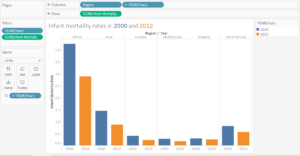This works with a handful of regions, but if you decide to expand the view to include country, things become messier.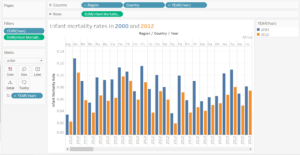2. An Un-stacked bar chart

By flipping the bar chart to be horizontal (easier to read country names) and un-stacking the two measures you can represent the difference in measure between those two time points, this works best when the change is consistent in direction but may change in magnitude.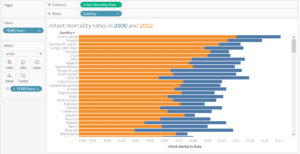Drag the dimension pill to the rows shelf, the measure pill to the columns shelf, the year to the filter (filter to the two time points you want to visualise) and also to colour. Click on the analysis drop-down menu at the top, un-tick aggregate measures, and un-tick the stack marks option.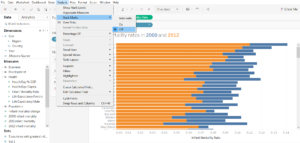3. The table calculation

Let’s create some table calculations to visualise the change as a single measure. Firstly, we’ll make table calculations for each of the years we want to compare – 2000 and 2012.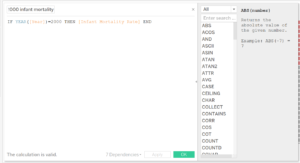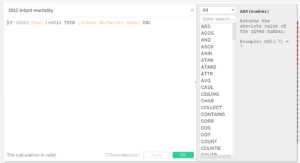Now we’ll use these calculations to give us the change.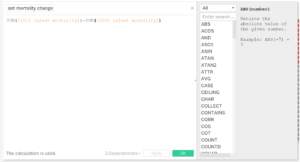It’s generally best to calculate by subtracting the earlier date from the later, as it is most intuitive for us to think of things going up or down over time.

Drag this newly created table calculation onto the columns shelf and the dimension onto the rows shelf.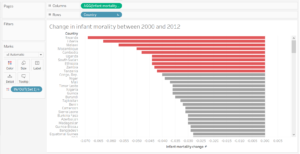To add a splash of colour to distinguish the top reductions we can create a set: right click on the measure and select create – set.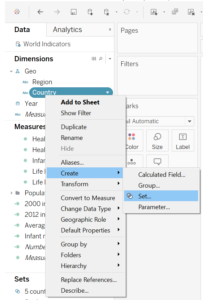Because the table calculation results in negative change, we need to create the set using the top 10 members of the inverse of the table calculation.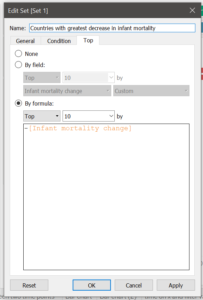Then drag the set onto colour.

4. The dumbbell chart

A really fun way to visualise the change between two time points is with a dumbell chart.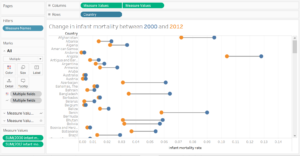To create a dumbell chart, start by dragging my dimension pill onto the rows field and then dragging the measure names pill onto filters and selecting only the two measures you want to include, in this case the table calculations for 2000 and 2012 that we created earlier.

Then drag measure values onto the columns shelf, and change the mark type to circles and change measure names to colour.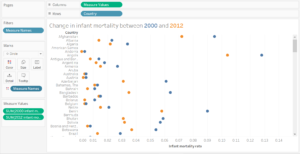Hold control drag the measure values pill next to itself to create a second axis, right click and select dual axis. Right click, on the axis header and synchronise the axis.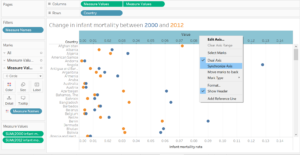On the marks card, select the top measure value and change the mark type to a line and then change measure value from colour to path.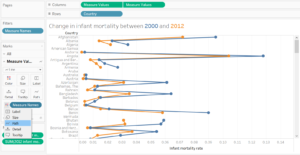Right click on one of your axis and un-tick show header to hide the extra axis.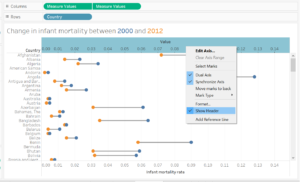5. Percentile change scatterplot

I love these, though they can be quite confusing.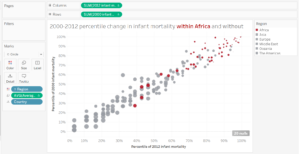A mark’s position up and along the axis represent its relative level within each of the measures, an additional percentile calculation has been added to negate the clustering of marks that often occurs; as such this chart only serves to show a marks relative place compared to other marks, rather than any absolute values.

Use the calculated fields for 2000 and 2012 that you created earlier as your rows and columns, and apply the following table calculation to each.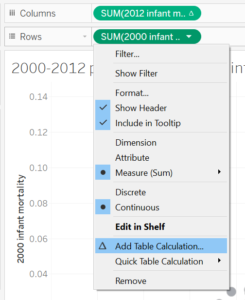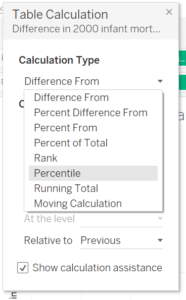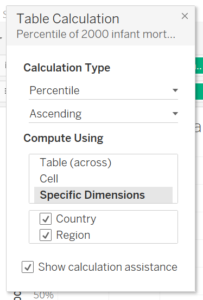Make sure to do this to both measures.

You can use another measure to influence the size of the marks, and colour by a dimension (as above) or  by a set, such as the one created earlier.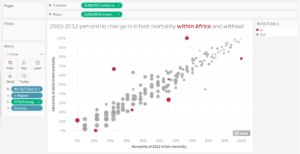Andrew Lehm